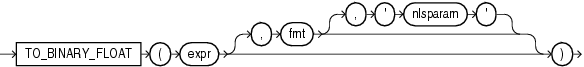TO_BINARY_FLOAT

SyntaxDescription of the illustration to_binary_float.gif

Purpose

TO_BINARY_FLOAT returns a single-precision floating-point number.

• expr can be a character string or a numeric value of type NUMBER, BINARY_FLOAT, or BINARY_DOUBLE. If expr is BINARY_FLOAT, then the function returns expr.

• The optional 'fmt' and 'nlsparam' arguments are valid only if expr is a character string. They serve the same purpose as for the TO_CHAR (number) function.

• The incase-sensitive string 'INF' is converted to positive infinity.

• The incase-sensitive string '-INF' is converted to negative identity.

• The incase-sensitive string 'NaN' is converted to NaN (not a number).

You cannot use a floating-point number format element (F, f, D, or d) in a character string expr.

Conversions from character strings or NUMBER to BINARY_FLOAT can be inexact, because the NUMBER and character types use decimal precision to represent the numeric value and BINARY_FLOAT uses binary precision.

Conversions from BINARY_DOUBLE to BINARY_FLOAT are inexact if the BINARY_DOUBLE value uses more bits of precision than supported by the BINARY_FLOAT.

Examples

Using table float_point_demo created for TO_BINARY_DOUBLE, the following example converts a value of data type NUMBER to a value of data type BINARY_FLOAT:

SELECT dec_num, TO_BINARY_FLOAT(dec_num)
FROM float_point_demo;

DEC_NUM TO_BINARY_FLOAT(DEC_NUM)
---------- ------------------------
1234.56               1.235E+003